# Anomalously Non-Anomalous Anomaly Detection Results

## The RX Anomaly Detector¶

In image processing, anomaly detectors are algorithms used to detect image pixels that are sufficiently different than other pixels in the same image (or within a local neighborhood of the pixel being evaluated). The RX anomaly detector  represents each pixel in an image as a point in an $N$-dimensional space, where $N$ is the number of bands in the image. The image background is assumed to be distributed as an $N$-dimensional Gaussian distribution with mean vector $\mathbf{\boldsymbol{\mu}}_{b}$ and covariance matrix $\boldsymbol{\Sigma}_{b}$. Each pixel is then compared to the image background by computing the squared Mahalanobis distance of the pixel from the background:

$$D_{M}^2(\mathbf{x})=(\mathbf{x}-\boldsymbol{\mu}_{b})^{T} \boldsymbol{\Sigma}_{b}^{-1}(\mathbf{x}-\boldsymbol{\mu}_{b})$$

Under the Gaussian assumption, as $D_M$ increases, the probability of associated values of $\mathbf{x}$ decreases. In other words, $\mathrm{p}(\mathbf{x})$ decreases monotonically with increasing $D_M(\mathbf{x})$. Therefore, an anomaly detection threshold can be placed on $D_M$ corresponding to a desired probability of exceedance. If instead of choosing a detector threshold we want to see where the "most anomalous" pixels are in the image, we can construct a new image, whose pixel intensities are scaled based on corresponding RX anomaly detector values.

## The Input Image¶

Shortly after I added an RX anomaly detector function to the Spectral Python package, I was asked for an example of how it is used so I decided to use the AVIRIS image chip that is used throughout the user's guide. This particular image, which has been widely studied , is a 145 $\times$ 145 pixel image chip containing 220 spectral bands collected over an agricultural area in Indiana. Before computing the RX scores, let's take a look at the image.

In :
%matplotlib  inline
import matplotlib.pyplot as plt
import numpy as np
import spectral as spy

spy.settings.show_progress = False
image = spy.open_image('92AV3C.lan')
(nrows, ncols, nbands) = data.shape

In :
spy.imshow(data, (30, 20, 10))
plt.title('Natural Color (RGB)');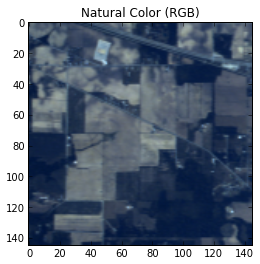In :
spy.imshow(data, (50, 20, 10))
plt.title('False Color (RG-NIR)');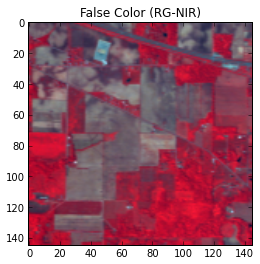The first image shows the RGB bands of the image, which renders the scene close to how it would look to the eye. The second is a false color image, where we have replaced the red band with a near infrared (NIR) band at approximately 870 nm.

## RX Anomaly Detector Output¶

Next, we'll compute and display the RX scores for the image. We could use a local, rolling window for computing background statistics but for this example, we'll just use the entire image chip to compute the background statistics.

In :
rximg = spy.rx(data)
spy.imshow(rximg);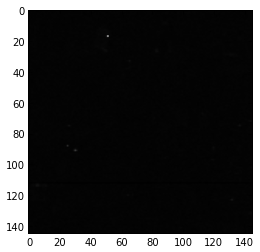In the RX image above, only a few bright spots (high RX scores) are visible because a linear gray-scale is used and the visible bright pixels have RX scores much higher than the rest of the image. If we want to see more detail within the RX image we need to either use a nonlinear color scale or - equivalently - scale the data nonlinearly. Let's take the $\mathrm{log}$ of the RX scores and see what additional details are presented.

In :
spy.imshow(np.log(rximg));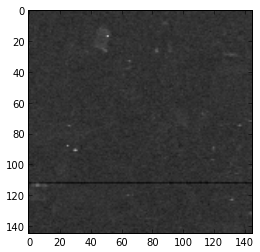## (Non)Anomalous Pixels¶

Transforming the RX scores to a nonlinear scale brings out some additional detail in the image but what is the black line near the bottom of the RX image? The display suggests that RX scores are consistently lower in that row of the image but there do not appear to be any corresponding features in the RGB or false color views shown above. Let's plot the RX scores of all rows for a single column and then for all pixels of the row in question.

In :
(r, c) = (112, 80)
plt.figure(figsize=(8, 8))
plt.subplot(2, 1, 1)
plt.plot(rximg[:, 80])
plt.xlabel('image row')
plt.ylabel('RX value')
plt.grid(1)
plt.title('RX scores in image column %d' % c)
plt.subplot(2, 1, 2)
plt.plot(rximg[112, :])
plt.xlabel('image column')
plt.ylabel('RX value')
plt.grid(1)
plt.title('RX scores in image row %d' % r);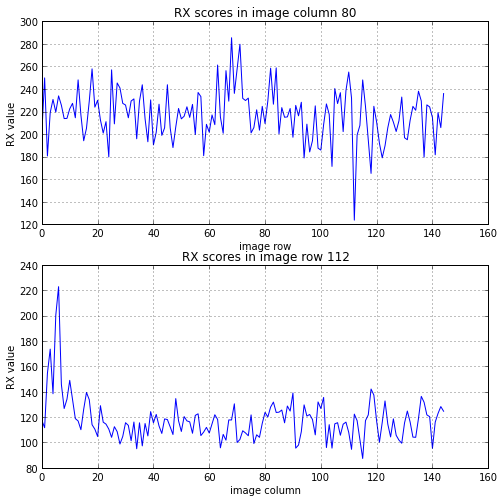The RX scores in row 112 (counting from zero) are significantly lower than the other rows. Let's calculate the mean RX score in row 112 and then calculate the probability that the RX score of a random pixel (under the assumption of a Gaussian background) would be less than or equal to that value. For a 220-band image, the RX score (squared Mahalanobis distance) is distributed as $\chi_{220}^2$ (a chi squared distribution with 220 degrees of freedom).

In :
from scipy.stats import chi2
row_mean = np.mean(rximg[112, :])
P = chi2.cdf(row_mean, nbands)
print 'Mean RX value in row 112 =', row_mean
print 'P(RX <= %f) = %e' % (row_mean, P)

Mean RX value in row 112 = 117.748294213
P(RX <= 117.748294) = 1.760758e-09


Clearly, there is something abnormal here (no pun intended) for so many RX values to be so low but what could be causing this to occur? The RGB and false color image displays do not indicate anything obvious in that row but each of those views only shows three of the 220 spectral bands from the source image. Perhaps the cause of this phenomenon lies in one of the bands that we haven't seen. Let's render all bands for the column of pixels that we plotted above.

In :
plt.imshow(data[:, 80, :])
plt.ylabel('image row')
plt.xlabel('band number');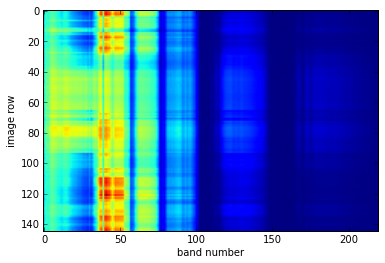Visually comparing image row 112 to others in the figure above, there doesn't appear to be anything unique to row 112. Next, let's plot the first few bands of one of the low-RX pixels, along with the two pixels directly above and below it.

In :
plt.figure(figsize=(8, 4))
plt.plot(image.bands.centers[:20], data[r, c, :20], 'r.-', label='low RX pixel')
plt.plot(image.bands.centers[:20], data[r - 1, c, :20], 'g.:', label='pixel above')
plt.plot(image.bands.centers[:20], data[r + 1, c, :20], 'b.:', label='pixel below')
plt.xlim(image.bands.centers, image.bands.centers)
plt.grid(1)
plt.xlabel('wavelength (nm)')
plt.legend(loc='best');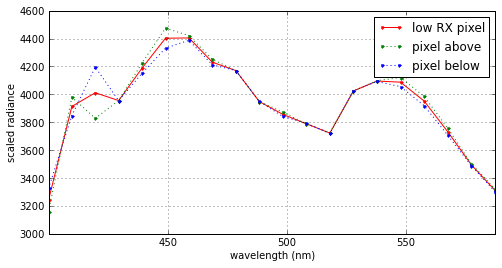There doesn't appear to be anything unusual with the low-RX pixel relative to the adjacent pixels. For most bands, the low-RX pixel has radiance values between the other two pixels values.........Actually, it appears to have values that are exactly at the midpoint between the adjacent pixel values.

Could it be that the low RX scores are because row 112 is actually an average of the rows above and below it? Let's calculate the maximum absolute difference between band values of the pixel in row 112 and values averaged from the pixels in the rows immediately above and below it.

In :
avg = (data[r - 1, c] + data[r + 1, c]) / 2.0
np.max(np.abs(avg - data[r, c]))

Out:
0.5

A maximum difference of 0.5 is consistent with truncation due to integer division (the image data file contains integer values of scaled radiance). So we can conclude that row 112 is indeed an average of the two adjacent rows.

Why would pixels be averaged this way? There are several possibilities but two of the most common are that there was either a detector failure (the data for pixels in the row were corrupted or not collected) or a disk write failure. Rather than discard an entire image or have an unsightly artifact running through it, the band values of the missing/corrupted line are linearly interpolated (averaged) from the adjacent lines.

## Explanation of Low RX Values¶

The RX values of the averaged pixels are lower because averaging pixels brings them closer to the background mean. But we can also quantify the expected reduction in RX values.

The problem is analogous to estimating the mean of a Gaussian distribution. Given a Gaussian distribution with unknown mean ($\mu$) and variance ($\sigma^2$), we can compute an estimate of the mean ($\bar{x}$) by taking the average of $N$ samples. Since $\bar{x}$ is computed from random values, it is also a random quantity, with a mean value of $\mu_{\bar{x}}=\mu$ and a variance $\sigma_{\bar{x}}^2=\sigma^2/N$. The two limiting cases are $N=1$ and the limit as $N\rightarrow\infty$. When $N=1$, $\bar{x}$ is equal to a single instance drawn from the distribution so $\sigma_{\bar{x}}^2=\sigma^2$. As $N\rightarrow\infty$, $\bar{x}$ is computed from the entire distribution so $\sigma_{\bar{x}}^2\rightarrow0$.

Averaging pixels prior to the RX calculation is similar except instead of computing the mean squared deviation (variance), we are computing the sum of squared deviations (squared Mahalanobis distance). The $\chi_k^2$ distribution has mean value $k$. By averaging $N$ values prior to computing the RX score, we reduce the mean value by a factor of $N$ so the expected RX score for an average of $N$ background pixels is $k/N$. To show this, we will average varying numbers of pixels from the image and compare the corresponding RX scores with the corresponding Expected mean RX scores for a 220-dimensional Gaussian distribution.

In :
(r, c) = (113, 80)
stats = spy.calc_stats(data)
nvals = range(1, 11)
rxvals = [spy.rx(np.mean(data[r: r + n, c, :], axis=0), background=stats)
for n in nvals]
chi2vals = [220. / i for i in nvals]
plt.plot(nvals, rxvals, 'o-', label='averaged image pixels')
plt.hold(1)
plt.plot(nvals, chi2vals, '.r--', label='expected value for 220-band Gaussian')
plt.grid(1)
plt.xlabel('number of pixels averaged')
plt.ylabel('RX value')
plt.gca().set_title('RX values for averaged spectra')
plt.legend(loc='best');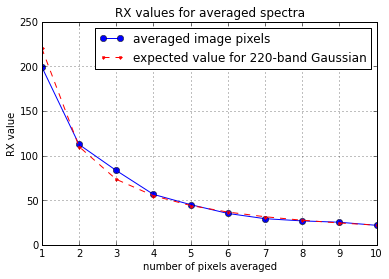It is worth emphasizing that the theoretical expected RX values (red series above) are independent of any image data. They are solely dependent on the number of image bands and the assumption that the data are distributed as a Gaussian. While the theoretical curve (which assumes random samples) tends to zero only in the limit as $N\rightarrow\infty$, The RX value for averaged image pixels will be identically zero when all image pixels are averaged, since the average is then identical to the background mean:

In :
spy.rx(stats.mean, background=stats)

Out:
0.0

 Reed, I.S. and Yu, X., "Adaptive multiple-band CFAR detection of an optical pattern with unknown spectral distribution," IEEE Trans. Acoust., Speech, Signal Processing, vol. 38, pp. 1760-1770, Oct. 1990.

 Landgrebe, D. Multispectral data analysis: A signal theory perspective. School of Electr. Comput. Eng., Purdue Univ., West Lafayette, IN (1998).